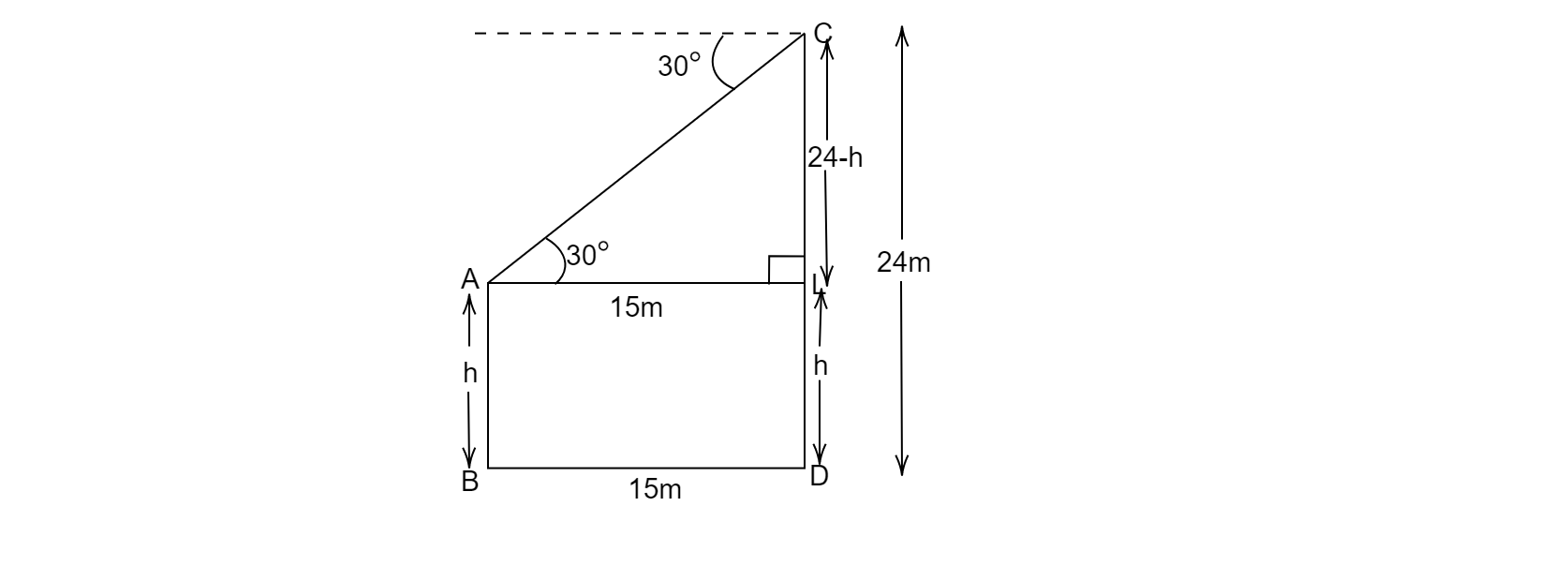# The horizantol distance between two poles is 15 cm. The angle of depression of the top of first pole as seen from the top of second pole is 30$^{o}$. If the height of the second pole is 24 cm, find the height of the first pole. [use $\pi =\frac{22}{7}$] .

Given: The horizontal distance between two poles is$=15\ cm$
The angle of depression of the top of first pole as seen from the top of second pole is $30^{o}$. The height of the second pole is 24 cm

What to do: To find the height of the first pole.

Solution:Let AB and CD be the two poles,

height of the first pole=24m

Let us say the height of first pole $AB=h$

Distance between the two poles, $BD = 15\ m$

$AL= BD = 15 m\ and\ AB=DL=h , CL = CD-DL$

$\vartriangle CAL$,

$tan30^{o}=\frac{CL}{AL}$

$\Rightarrow \frac{1}{\sqrt{3}} =\frac{24-h}{15}$

$\Rightarrow 24-h=\frac{15}{\sqrt{3}}$

$\Rightarrow h=24-\ \frac{15}{\sqrt{3}}$

$\Rightarrow h=24-5\sqrt{3}$

$\Rightarrow h=24-5\times 1.732$

$h=15.34\ m$

$\therefore$ The height of second pole is 15.34 m.